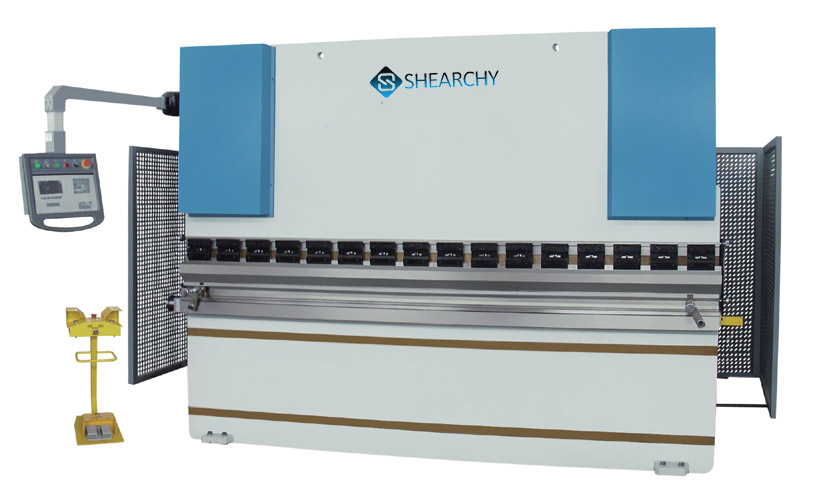In the process of CNC Press Brake Bending, the layout of bending process is an important factor that affects the efficiency of plate bending machine. The working procedure of common hydraulic bendingmachine is mainly prepared by hand. It’s low efficiency and high cost. Therefore, there is a bendingprocess planning algorithm based on differential evolution algorithm to improve the efficiency.

## Algorithm and Realization of CNC Press Brake Bending Processing

### Step 1: research on machining types and key parameters

• The calculation formula of key parameters. Such as: bending force and spring back, the basis of selecting upper and lower bending dies.
• And determine the criterion of bending priority.

### Step 2: interference collision detection algorithm

• In the process of metal bend, establish the brake press coordinate system and each key point of bending.
• Through the position relationship among several coordinate systems, obtain the position of bending key points in the machinery coordinate system. Compare with the dimensions of work piece and machine tools to determine whether there will be interference at this time.
• The algorithm ensures that the work piece itself does not collide, the workpiece does not collide with the upper and lower dies, and the workpiece does not collide with the machine tools. Prepare for the following process layout optimization algorithm.

#### Step 3: process layout optimization algorithm based on differential evolution algorithm

• In the case of no interference collision, give comprehensive consideration. For example, the number of turning, the number of press brake punch and die replacement, the moving time of each metalbend and other factors.
• The differential evolution algorithm can solve the multi-objective optimization problems. In order to minimize the total processing time, propose a process optimization algorithm. Then, this can find a reasonable bending process and improve the efficiency of platebending.

#### Step 4: design simulation test program

• According to the size parameters of bending work pieces, Cnc press brake bending machine tool and molds, we can simulate and test the bendprocess. So verify the correctness of interference detection algorithm and planning algorithm by comparing the results of simulation test and algorithm operation.